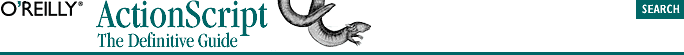home | O'Reilly's CD bookshelfs | FreeBSD | Linux | Cisco | Cisco Exam## 11.10. Multidimensional Arrays

The simplest type of multidimensional array is a two-dimensional array, in which elements are organized conceptually into a grid of rows and columns -- the rows are the first dimension of the array, and the columns are the second.

Let's consider how a two-dimensional array works with a practical example. Suppose we're processing an order that contains three products, each with a quantity and a price. We want to simulate a spreadsheet with three rows (one for each product) and two columns (one for the quantity and one for the price). We create a separate array for each row, treating the elements as columns:

```var row1 = [6, 2.99];   // Quantity 6, Price 2.99
var row2 = [4, 9.99];   // Quantity 4, Price 9.99
var row3 = [1, 59.99];  // Quantity 1, Price 59.99```

Next, we place the rows into a container array named spreadsheet:

`var spreadsheet = [row1, row2, row3];`

Now we can find the total cost of the order by multiplying the quantity and price of each row and adding them all together. We access a two-dimensional array's elements using two indexes (one for the row and one for the column). The expression spreadsheet, for example, represents the first row's two-column array. Hence, to access the second column in the first row of spreadsheet, we use spreadsheet:

```// Create a variable to store the total cost of the order
var total;

// Now find the cost of the order. For each row, multiply the columns
// together, and add that to the total.
for (var i = 0; i < spreadsheet.length; i++) {